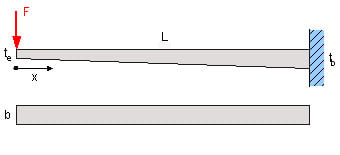### Tapered Snap Fit Beam Bending Equation and Calculator

Deflection equation and calculator for a tapered beam used in typical plastics design.Deflection EquationsWhere:

 E = Modulus of Elasticity psi (N/m2) F = Load lbs (N) s = Stress at the cross-section being evaluated Lbs/in2 (N/m2) d = Deflection inches (m)